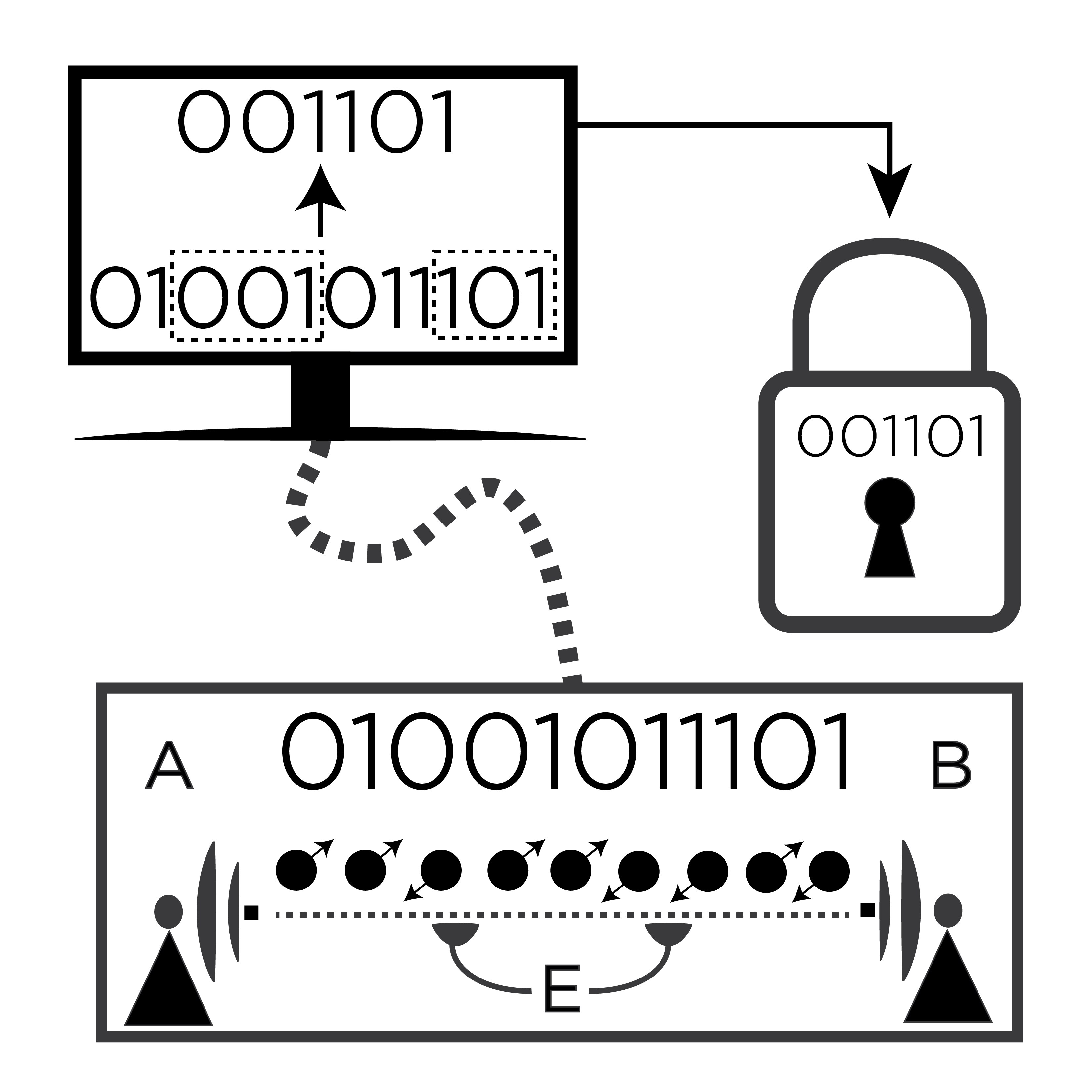# Numerical approach for unstructured quantum key distribution### Abstract

Quantum key distribution (QKD) allows for communication with security guaranteed by quantum theory. The main theoretical problem in QKD is to calculate the secret key rate for a given protocol. Analytical formulas are known for protocols with symmetries, since symmetry simplifies the analysis. However, experimental imperfections break symmetries, hence the effect of imperfections on key rates is difficult to estimate. Furthermore, it is an interesting question whether (intentionally) asymmetric protocols could outperform symmetric ones. Here we develop a robust numerical approach for calculating the key rate for arbitrary discrete-variable QKD protocols. Ultimately this will allow researchers to study ‘unstructured’ protocols, that is, those that lack symmetry. Our approach relies on transforming the key rate calculation to the dual optimization problem, which markedly reduces the number of parameters and hence the calculation time. We illustrate our method by investigating some unstructured protocols for which the key rate was previously unknown.

Type
Publication
Nature Communications, 7 11712
Date